Communication Technology Error Detection/Correction      There are many cases where we have to seriously consider 'Error'. Actually in almost every data processing and communications error handling is one of the most critical part of the process. Several common examples of the case where some form of error handling is used are as follows : Data Storage and read on Memory, Harddisk, CD etc Serial Communication (RS 232) IP Communication Most of Wireless Communication (e.g, Mobile Communication like WCDMA, LTE etc) You would realize that most of digital data processing or communication would have not be realized if there is no mechism for error handling (error detection and correction)   Followings are the topics that will be dealt in this page. What is Error ? What is Error Detection ? What is Error Correction ? Error Detection/Correction Techniques What is done if the reciever find the error ?     What is Error ?   First let's think of the definition of 'Error'. What is 'Error' ? It is simple. Error can be defined as 'any difference between the transmitted data and received data'. This may sound specific only to communication. In case of data storage, you can define Error as 'any difference between the data being sent for writing and the data really written on a media (e.g, Hard Disk, Memory or CD).'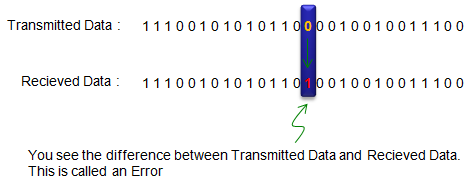What is Error Detection ?   Once Error happened, we need to have some mechanism to figure out 'whether there exists any error' or 'exactly where is the error occured'. This mechanism of finding the existence of error is called 'Error Detection'. If we know the exact contents of transmitted data, it would be very simple to find out the location of errors. Just simply by comparing the transmitted data and received data, we can easily find the location of errors. However in realty, we don't know what is the transmitted data (original data). So we have to figure out the location of errors only from the received data. This is why Error detection is not so simple.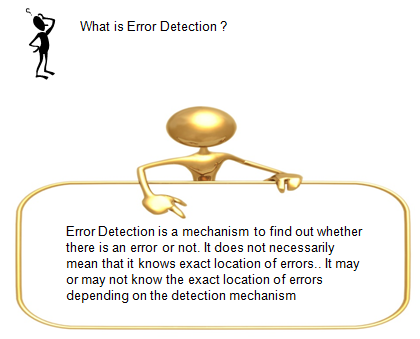There are roughly two types of Error Detection as follows. A mechanism that tells on the existence of error, but does not tells on the location of errors. A mechanism that tells on both the existence of error and the location of errors. Of course, the second type is better in most case since it will give a chance for error recovery. But in most cases, this second type tend to be more complicated to be implemented or requires more overhead for data transfer. This is why the first type of mechanism is still being used in many applications.     What is Error Correction ?   What is Error Correction ? The definition is simple.. (only Algorithm is complicated :). Definition of Error Correction is to fixing the error bit (data) to normal bit (data).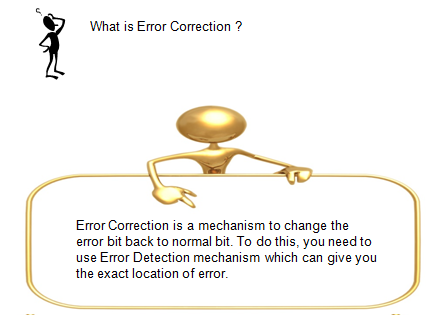Error Detection/Correction Techniques   Whatever you do, there is no way to prevent any error. The only thing you can do for those errors is to come out with the various methods of detecting and fixing those errors. For this detection/correction, method we need to go through roughly following procedures.   i) Convert (organize) the original data into a special structure in such a way that the reciever can easily detect the error. (This happens on Transmitter side). ii) Trasmit the processed data. iii) Receive the data. iv) Check if there is any error in the received data. This is called Error Detection and happens in the reciever side. v) If errors are found in step iv), ask for retransmission (ARQ case) or try to recover(fix) the error (FEC or HARQ case). This happens in reciever side.   When we talk about Error Detection or Correction (step v), we normally explain about step i) as well because the error detection/correction methods varies depending on step i).   In this section, I will briefly explain about several most common methods for step i) and iv), v) that are used for data communication.     < Parity Check >   If you convert data into binary stream and count the number of '1' in the data, it would be only one of two cases.. the total count of '1' is Even or Odd. In Parity Check method, transmitter reorganize the original data so that the total number of '1' is only Even (Even Parity) or only Odd (Odd Parity). Following is the example process for 'Even Parity' algorithm. This is very simple and still being used in some simple data communication like RS 232 Serial communication. But this is detection only algorithm and it cannot correct error. Even for detection, there are many cases where this algorithm fail to detect errors. (Try think of those cases where this algorithm fails)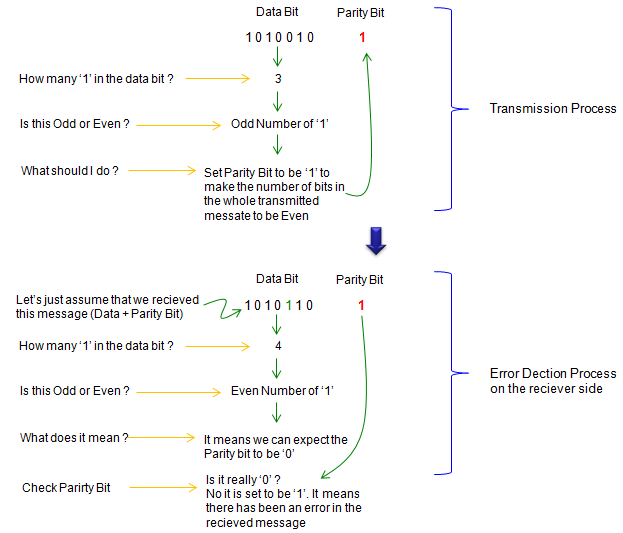< Two Dimensional Parity Check >   The concept and method of Two Dimensional Parity check is almost the same as the simple parity check explained above. The only difference is that in this algorithm we convert the data into a 2 dimensional array and apply parity bits horizontal and vertical direction as illustrated below.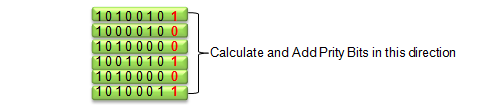< Checksum >   In Checksum algorithm, we calculate a special number called 'checksum' value from the original data and add the checksum data to the transmitted data. When the reciever get the data, it calculate the checksum value from the received data and compares the calculated value with the received checksum value. Definately it looks more complicated than Parity Algorithm, but it is more robust in finding errors. This is also detection only algorithm. Most common application of this algorithm is IP transmission. If you decode any IP packet decoded in wireshark, you would see the checksum field in every packet.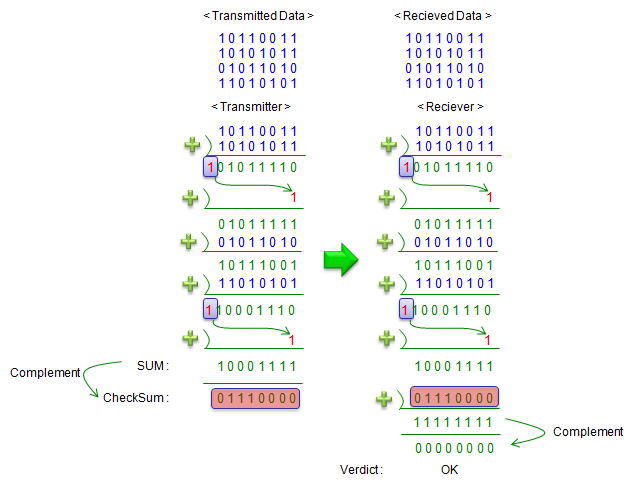< Cyclic Redundancy Error (CRC) >   As in CheckSum algorithm, in CRC algorithm .. the transmitter calculate special number called CRC bits as shown in the following example and attach the value at the end of the original data and send it.  When the reciever get the data, it calculate the CRC value from the received data and compares the calculated value with the CRC value.   Overall procedure can be summarized as shown below.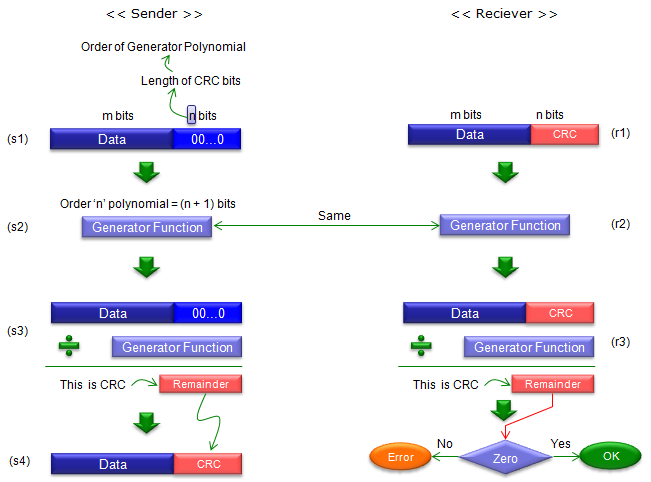The value m and n varies depending on cases. Normally, a standards for a specific communication technolgy (e.g, 3GPP in case of WCDMA, LTE) predefines these value. One of the critical thing is how you determine "Generator Function" (sometimes called 'Divisor'). This would require deep mathematical knowledge on Field Theory.. but fortunately you don't have to pull your hair trying to determine this because the international standard in your industry would specify these functions :)   Next important and confusing step would be step s3. One example is as follows. (For more practical example using less scary mathematics -:), see CRC page)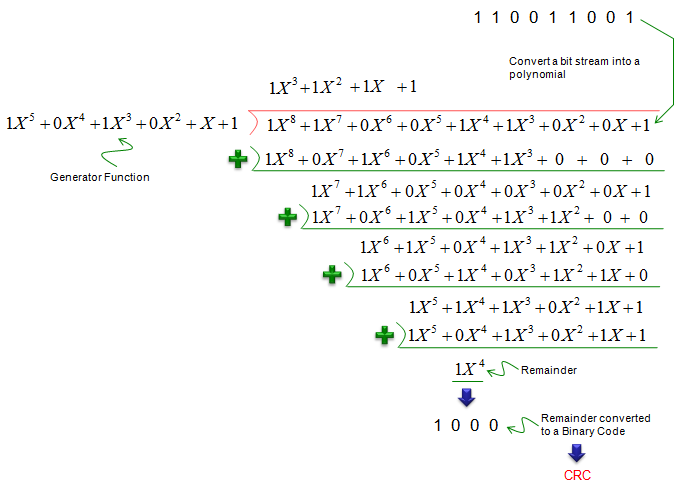Here goes a couple of example of Generator Function that is used in various communication system.   The Generator Function that is used in LTE is shown below. (3GPP 36.211 5.1.1 CRC calculation)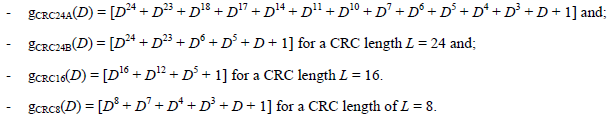The Generator Function that is used in UMTS is shown below. (3GPP 25.212 4.2.1.1 CRC Calculation)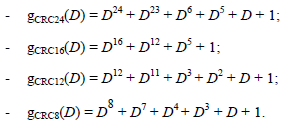This may be the algorithm that is most commonly used in wireless communication.     What is done if the reciever find the error ?   What a system (two communicating parties) do if the reciever find (detect) an error ? We see roughly three different mode of operations depending on what kind of error handling mechanism the system uses as listed below. ARQ (Automatic Repeat reQuest) FEC (Forward Error Correction) HARQ (Hybrid ARQ) In ARQ, the reciever ask for retransmission of exactly the same data by sending Nack or by skipping any Ack to the transmitter. If the transmitter does get Nack or does not get Ack within a certain time frame, it automatically retransmit the exactly the same data that was transmitted before. The advantage of this mechanism would be that it is simple to implement and it only has to implement error detection algorithm and does not implement the error correction algorithm. The disadvantage would be that the transmiter would have to send the same data so many times if the channel condition is bad and the reciever keep detecting errors. Most common examples for this mechanism would be IP data or RLC layer transmission in WCDMA, LTE.   In FEC, transmitter encode the data before transmitssion in such a way that the reciever not only detect the error but also recover the error unless the amount of the error exceeds a certain level. In this case, the reciever does not request retransmission, in stead it recover (correct) errors from the redundancy bits and specially designed structure contained in the received data itself. The advantage of this mechanis is obvious. It is that we don't need retransmission even when there is error. The disadvantage is that it can recover the error up to only a certain level, if there is more errors exceeding the specific level it is impossible to recover error. In order to enable more capability of error correction, we have to put more redundency bits in the transmitted data, meaning it would increase overhead.   HARQ has the property of both ARQ and FEC. It is a kind of Hybrid algorithm and it is where the name come from. It is Hybrid of ARQ and FEC. When the reciever got a data with error, furst it tries to recover (correct) the error with FEC algorithm. If the error correct was successful, it send Ack to the sender. If it fails, it sends Nack to sender. When the sender got the Nack (or no response) from the reciever, it retransmit the data but when it retransmit the data, it usually send a little bit different sets of the original data (we call it 'different redundency version') and the reciever combines the previous copy and the retransmitted copy to increase probability of error recovery. The advantage of this algorithm is that it adopts the advantage of both ARQ and FEC and reducing the disadvantage of FEC and ARQ. But this would be more complicated to implement than simple ARQ and FEC. One common example of this mechanism is Physical layer communication in HSDPA and LTE. For further details, refer to LTE HARQ page.